[TOC]

# 1. Introduction

rearanging array of size N into ascending order
test client code: `Insertion.sort(a);`

sort any datatype

### callback

callback = reference to executable code
i.e. passing functions as argument to sort() method
sort() function calls object's `compareTo()` method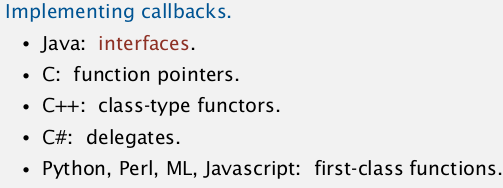→ implement the `Comparable` interface:

```    public class XX implements Comparable<XX>{
...
}
```

the interface:

```    public interface Comparable<Item>{
public int compareTo(Item that);
}
```

compareTo():
return -1 (if this<that)/+1/0; needs a total order. → in the sort() implementation:
has not dependencies on type of data.

```    public static void sort(Comparable[] a){
if(a[i].compareTo(a[j])>0)...
}
```

### helper functions

• less

private static boolean less(Comparable v, Comparable u){ returnv.compareTo(u)<0; }

• exch

private void exch(Comparable[] a, int i, int j) { Comparable swap = a[i]; a[i] = a[j]; a[j] = swap; }

• isSorted

test if sorted if algo passes the test using only less ant swap, then it's correct.

# 2. Selection Sort

Idea: each time: find the minimum from the remaining items. a[min] is the smallest element to right of a[i] ⇒ swap a[i] and a[min] (elements to left of i are sorted)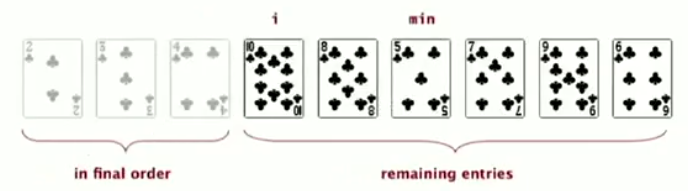## invariants

• entries to the left of i are in sorted order, and are fixed (in final position) ever since
• no entry to the right of i is smaller than any entry to the left of i

## implementation

```public class SelectionSort extends AbstractSorting{
//...
public static void sort(Comparable[] a){
for(int i = 0; i<a.length; i++){
int min = i;
for(int j = i+1; j<a.length; j++)
if(less(a[j],a[min]))
min = j;
exch(a,min,i);
}
}
}
```

## analysis

proposition: selection sort uses N-1 + N-2 + ... + 1 = ~N^2/2 compares, and N exchanges. → quadratic time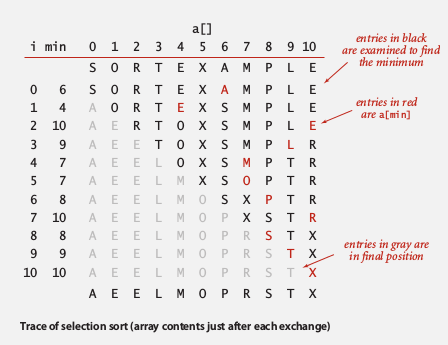• insensitive to input: quadratic time even if input is already sorted.
• data movement is minimum: linear time of exchanges (every exchange puts an item to its final position)

# 3. Insertion sort

quite different performance characteritics than selection sort.

Idea: In iteration i: move all entries larger than a[i] to its left.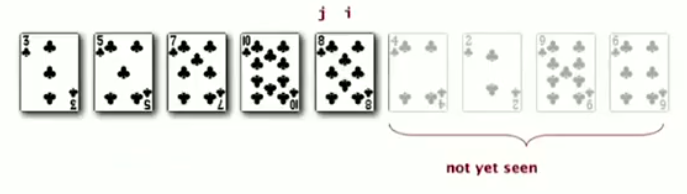## invariants

• entries to the left of i are in ascending order (but not in final position)
• entries to the right of i are not yet been seen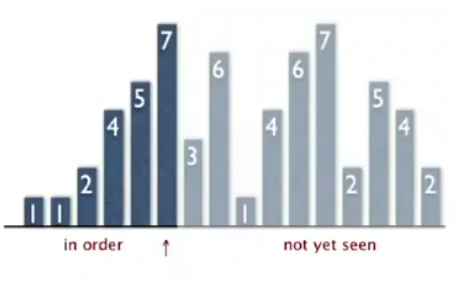## implementation

```publc class InsertionSorting extends AbstractSorting{
public static void sort(Comparable[] a){
for(int i=1; i<a.length; i++)
for(int j=i; j>0; j--){
if(less(a[j],a[j-1]))
exch(a,j,j-1);
else break;
}
}
//...
}
```

## analysis

proposition (average case):
(the performance on averagefor randomly sorted array )proof: expect each entry to move halfway back### best case and worst case

best case if array already sorted min ascending order: N-1 compares, 0 exchanges.

worst case if array sorted in descending order: every element goes all the way back → 1/2N^2 compares, 1/2N^2 exchanges

### partially sorted arrays

def." inversion" an inversion is a pair of entries that are out of order.

def. "partially sorted"
An array is called partially sorted if the number of inversions is <= cN. *

proposition.
Insertion sort runs in linear time for partially sorted array. proof. number of exchanges = number of inversions. number of compares = number of exchanges + N-1

# 4. Shell Sort

First non-trival sorting methode: an improvement of insertion sort.

def. "h-sorted array"
an array is h-sorted if every h-interleaved subarray is sorted. (h=1: just a sorted array)Idea: move entries >1 position at a time by h-sorting the array, then decrease h.

use decreasing sequences of value h: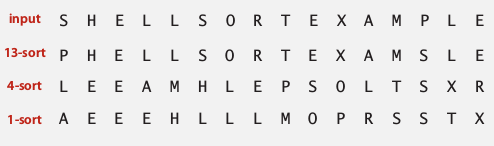## implementation

How to h -sort
simply insertion sort with stride length=h.
why insertion sort:
for big h: small subarray
for small h: nearly in order

proposition
A g-sorted array remains g-sorted after h-sorting it.(subtle to prove...)

which sequence of h to use `3x+1` sequence proposed by Knuth. 1,4,13,40....

```public class ShellSort extends AbstractSort{
public static void sort(Comparable[] a){
int h = 1, N=a.length;
while(h<N/3) h = h*3+1;//find the beginning h (N>h>N/3)
while(h>=1){//performs h-sort
for(int i= h;i<N;i+=h)
for(int j = i;j-h>=0;j-=h)
if( less(a[j],a[j-h]) )
exch(a,j,j-h)
else break;
h = h/3;
}
}
//...
private static boolean isHsorted(Comparable[] a, int h) {
for (int i = h; i < a.length; i++)
if (less(a[i], a[i-h])) return false;
return true;
}
}
```

## analysis

proposition (for worst case )→ better than quadratic time !

property (found in practice)

# of compares < Cte * N * (# of h used )

→ #compares < NlgN * Cte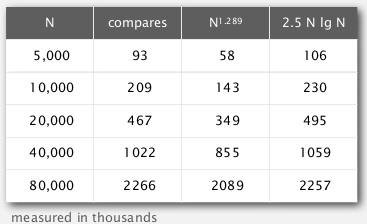*accurate model has not been discovered *

(所以shellsort在实际使用中几乎和快速排序一样快! — 尽管没有数学证明来保证)

why we are interested in shell sort useful in practice:

• fast for medium sized arrays (beat even the classical sophistiated algorithms)
• tiny code volumn (used in embeded systems)

lead to interesting questions for 50 years:

• asymptotic growth rate ?
• best sequence of h ?
• average case performance ?

# 5. shuffling

## shuffle array using sort

one way to shuffle an array:

1. for each array entry, generate a random real number
2. sort the array of real numbers
3. ⇒ the original array is shuffled !

proposition
this shuffle sort produces a uniformly random permutation of input array
drawback: cost for sorting...

Goal: get uniformly random permutation in linear time.

## Knuth shuffle

algo:

for i = [0,N):
- r = rand( [0~i] ) or rand( [i, N-1] )
- swap a[r] and a[i]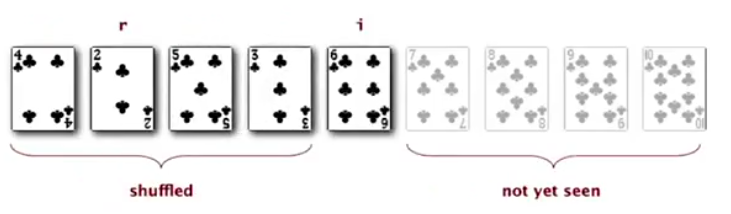implementation:

```public static void shuffle(Object[] a){
for(int i=0;i<a.length;i++){
int r = StdRandom.uniform(i+1);
exch(a,r,i);
}
}
```

proposition
Knuth algo produces an uniformly random permutation of input array.

proof.
Sufficient to prove that, for card i and position j, the proba(card i comes to position j) = 1/N.

1. if i<=j, P = 1/j * j/(j+1) * (j+1)/(j+2) * ... * (N-1)/N
2. if j<i, P = 1/i * i/(i+1) * (i+1)/(i+2) * ... * (N-1)/N

CQFD.

## example: online poker

https://www.cigital.com/papers/download/developer_gambling.php ←那个扑克网站已经被黑出翔了...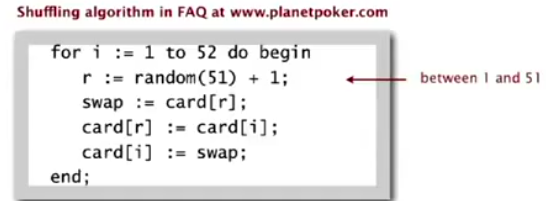bugs:

1. r never get 52 (52th card never moved)
2. r = rand(N) instead of rand(0~i), → shuffle not uniform
3. random() uses 32bit seed: only 2^32 possible shuffles, 2^32<52!
4. seed = millisec from midnight, ~86*10^6 suffles

# 6. Convex Hull

application of sorting for the field of computational geometry.

## convex hull

smallest polygoneenclosing all N points.• input: N points
• output: sequence of vertices in counterclockwise (ccw) order.

application: robot motion planning; farest pair.

geometric properties:

• can traverse convex hull by making only ccw turns
• let p be the point with lowest y-coord, wrt p, vertices appear in increasing order of polar angle.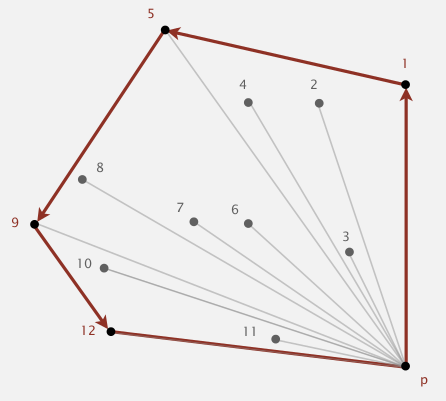## Algo

Graham scan algorithm:
* choose p with smallest y coord
* sort points by polar angle with p
* consider points in order (stack is used), discard unless creates a ccw turn.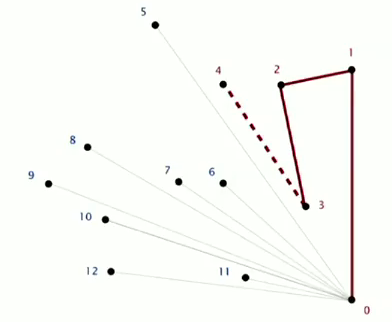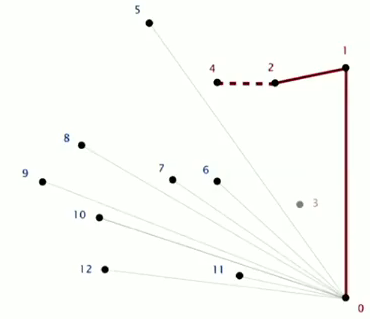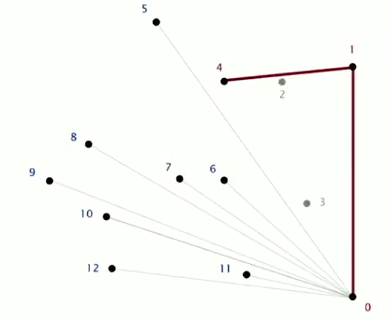CCW
given three points a b c, returns if a→b→c is a CCW turn.(assumption: no 3 points on a line)
⇒ calculate cross product of ab and bc ⇒ determinants!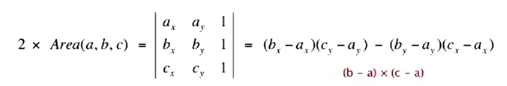area>0 ⇔ CCW

## implementation

```public class Point2D{
private double x,y;
public static boolean ccw(Point2D a,Point2D b,Point2D c){
double area2 = (b.x-a.x)*(c.y-a.y) - (b.y-a.y)*(c.x-a.x);
return area2>0;
}

}
```

convex hull:

```public static Stack<Point2D> GrahamScan(Point2D[] p){
//* assumes that points are sorted by polar angle in p[]
Stack<Point2D> hull = new Stack<Point2D>();
hull.push(p);
hull.push(p);
for(int i=2;i<p.length;i++){
Point2D b = hull.pop(), a = hull.peek(), c = p[i];
while(!Point2D.ccw(a,b,c)){
b = hull.pop();
a = hull.peek();
}
//now a,b,c makes a ccw turn:
hull.push(b);
hull.push(c);
}
}
```

running time: NlgN for sorting and linear for the rest.

#### Part 5 of series «Algorithms Princeton MOOC I»：

comments powered by Disqus